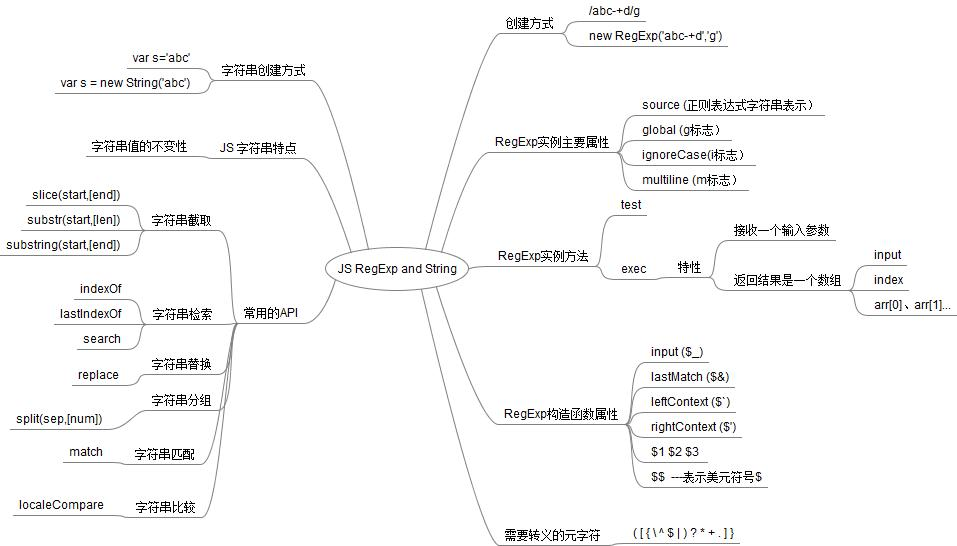# javascript正则表达式和字符串RegExp and String（二）```var s = 'abcd1234DCBA'; //推荐的创建字符串方式
var s1 = 'abcd1234DCBA';
var s2 = new String(s); // 通过构造方法创建字符串
var s3 = new String(s);
console.log(s===s1); //true 具有值类型的特性
console.log(s===s2); //false 基本类型和对象不相等
console.log(s2===s3); //false 不同的对象不相等
console.log(typeof s); // string
console.log(typeof s2); // object
//判断输入值是否是基本类型字符串
function isString(s) {
return typeof s === 'string'
}
console.log(isString(s)); //true
console.log(isString(s2)); //false
//判断输入值是否是字符串（基本类型+字符串对象形式）
function isString2(s) {
return s != null && typeof s.valueOf() === 'string';
}
console.log(isString2(s)); //true
console.log(isString2(s2)); //true```

```  var s = new String('abc');
var r = s.toUpperCase();
alert(s);  // abc  s本身是不会发生变化的

slice : String.slice(N1,N2) 这个就是我们常用的从指定的位置(N1)到指定的位置(N2)的字符串;
substring : String.substring(N1,N2) 这个就是我们常用的从指定的位置(N1)到指定的位置(N2)的字符串;
substr : String.substr(N1,N2) 这个就是我们常用的从指定的位置(N1)截取指定长度(N2)的字符串;

var s = '0123456789';
var r1 = s.substring(1); //123456789 第二个参数默认为字符length
var r2 = s.substring(1,5); //1234
var r3 = s.substring(1); //123456789 第二个参数默认为字符length
var r4 = s.substring(1,5); //1234
var r5 = s.substr(1); //123456789 第二个参数默认为字符length
var r6 = s.substr(2,5); //23456
var r7 = s.substr(2,100); //23456789 第二个参数大于字符length，不受影响

```var s = '0123abc401234';
console.log(s.indexOf('23')); // 2
console.log(s.lastIndexOf('23')); // 10
console.log(s.search(/[a-z]+/g)); // 4 检索出现字符的开始位置```

JS中一般调用replace方法来对字符串中某些字符的替换，该方法接收两个参数：
* 第一个参数是用来描述要被替换的子字符串，参数类型可以是字符串也可以是正则表达式。这里千万要注意，如果是字符串，则只会替换原字符串中第一个匹配的子串，而如果是未设置标识'g'的正则表达式，替换时得出的结果也是一样的，如果想替换所有匹配的子字符串，则必须传入带有标识g的正则表达式
* 第二个参数是用来描述替换值。参数类型可以是一个字符串，也可以是一个函数，还可以是包含特殊序列字符（RegExp的静态属性：−/&/‘/'/1..n/\$\$等）。

```var s = 'cat,bat,sat,fat';
var res = s.replace('at','NE');
console.log(res);//cNE,bat,sat,fat 只替换第一个匹配项
var res1 = s.replace(/at/,'NE');
console.log(res1); //cNE,bat,sat,fat 还是只替换第一个匹配项
var res2 = s.replace(/at/g,'NE');
console.log(res2); //cNE,bNE,sNE,fNE 替换所有的匹配项```

```var s = 'ab<name>cd';
// 模拟HTML对符合 < >进行转义
var res = s.replace(/[<>]/g,function(match,index,souStr) {
switch(match) {
case '<': return '<';
case '>': return '>';
}
});
console.log(res); // ab<name>cd```

```// 针对字符sou,将关键字key用{}扩起来
function strong(sou,key) {
var re = new RegExp('('+key+')','g');
return sou.replace(re,'{\$1}'); //\$1 第一个捕获组
}
console.log(strong(s,'at')); //c{at},b{at},s{at},f{at}
// 针对字符sou,将关键字key用{}扩起来
function strong2(sou,key) {
var re = new RegExp(key,'g');
return sou.replace(re,'{\$&}'); //\$& 匹配的字符串
}
console.log(strong2(s,'at')); //c{at},b{at},s{at},f{at}```

JS中采用split方法来对字符串进行分组，该方法可接收两个参数：
* 第一个参数表示分隔符，可以是字符串类型，也可以是RegExp对象。
* 第二个参数是可选的，表示接收组的数量，也就是返回结果数组的大 小。如果不指定该参数，表示返回所有组。

```var s = 'cat,bat,sat,fat';
var res = s.split(',');
console.log(res); //[ 'cat', 'bat', 'sat', 'fat' ]
var res2 = s.split(/,/);
console.log(res2); //[ 'cat', 'bat', 'sat', 'fat' ]
var res3 = s.split(/,/,2);
console.log(res3); //[ 'cat', 'bat' ] 只返回2组```

match() 方法将检索字符串 stringObject，以找到一个或多个与 regexp 匹配的文本。这个方法的行为在很大程度上有赖于 regexp 是否具有标志 g。

```var s = 'cat,bat,sat,fat';
var reg = /[a-z](at)/ ;
console.log(s.match(reg)); //[ 'cat', 'at', index: 0, input: 'cat,bat,sat,fat' ]
var res = s.match(/[a-z](at)/g);
console.log(res); //[ 'cat', 'bat', 'sat', 'fat' ]```

```var s1 = 'abc';
var s2 = 'bcd';
var s3 = new String('abc');
console.log(s1>s2); //true
console.log(s1==s3); //true 将s1与s3.toString()进行比较
console.log(s1.localeCompare(s2)); // -1 s1 小于 s2
console.log(s1.localeCompare(s3)); // 0 s1的值与s3相等```

Copyright © 2010 Gimoo.Net. All Rights Rreserved  京ICP备05050695号